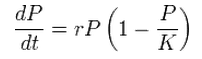I only spent a few hours on Nation Building this week but I have added dynamical growth. You can try it out here but it’s still far from a complete game.

For now I’m using a Logistic Function to model the growth of the human population and the ecology. A logistic growth function looks like this:• r is the growth rate
• P is the current population
• K is the carrying capacity (maximum population)

The left side of the equation represents the population increase after one growth cycle.

Each turn, for each tile, here’s how I use the equation in the game:

1. The human population grows according the above equation with r = 1.3 and K = the tile’s current Ecology
2. Ecology then decreases by the human population to represent the food being eaten
3. The ecology then grows with the same equation with r = 1.3 and K = 300 (an arbitrary max ecology)

On each tile, the humans are the black number and the ecology is the red number.I like this much better than the arbitrary growth model I was using previously but I still want to tweak it a lot. I’m thinking about having Population affect Ecology indirectly by modifying the Max Ecology (K) rather than the Ecology itself. This would simulate populations gradually erroding the soil.

Unrelated - I’ve started work on the interactions between bands. The game engine now identifies potential collisions and allows the bands to alter thier intended move. For now I just have the bands ‘bounce’ back to their current tile but in the future I’ll allows for fighting, trading, merging, etc.